Showing posts with label Measurements. Show all posts
Showing posts with label Measurements. Show all posts

## Differences Between Gravity Control and Spring Control

### Comparison of Gravity Control and Spring Control

Gravity control or Spring control both are comes under the category of "controlling torques" of an electrical measurement instrument.Before going have a comparison between gravity control and spring control let us have a look at why we need controlling torque in a measurement instrument?

1) Controlling torque produces a force equal and opposite to the deflecting force in order to make the deflection of pointer at a definite magnitude. If this system is absent then the pointer will swing beyond its final steady position for the given magnitude and deflection will become indefinite.

2) It brings the moving system back to zero position when the force which causes the movement of the moving system is removed. It will never come back to its zero position in the absence of controlling system. We have some advantages of spring control over gravity control.

#### Advantages of spring control | Disadvantages of gravity control

Gravity Control Spring Control
Adjustable small weight is used which produces
the controlling torque.
Two hair springs are used which exert controlling torque.
Controlling torque can be varied. Controlling torque is fixed.
The performance is not temperature dependent. The performance is temperature dependent.
The scale is nonuniform. The scale is uniform.
The controlling torque is proportional to sinA (A is angle). The controlling torque is proportional to 0.
The readings can not be taken accurately. The readings can be taken very accurately.
The system must be used in vertical position only. The system need not be necessarily in vertical position.
Proper levelling is required as gravity control. The levelling is not required.
Simple, cheap but delicate. Simple, rigid but costlier compared to gravity control.
Rarely used for indicating and portable instruments. Very popularly used in most of the instruments.

Tags:
Controlling System: Gravity vs Spring Control
Advantages of spring control over gravity control
What is advantages of spring control over gravity control
What is gravity control,spring control?
Controlling torque in measuring instruments

## What is Creeping in Energy Meters ?

### Creeping in Energy Meters:

In some energy meters a slow but continuous rotation of the disc is obtained when the pressure coils are energized and there is no load current passing through the current coil i.e. current coil is not energized.The main reason for creeping is over-compensation the aluminium disk to over come the static friction of disk and another reason is over voltage across the shunt magnet.Due to this creeping consumers suffers from high tariff. This may be due to incorrect friction compensation to vibration, to stray magnetic field or to the fact that the supply voltage is in excess of the normal voltage. This unwanted effect is called as "creeping in energy meter".

Read Here : How Energy Meter Works?

#### How to reduce creeping error in energy meter?

To prevent such creeping of the meter two holes or slots are cut in the disc on opposite sides of the spindle. The disc tends to remain stationary when one of the holes comes under one of the poles of the shunt magnet. In some cases a small piece of iron wire is attached to the edge of the disc. The force of attraction of the brake magnet upon this iron wire is sufficient to prevent the creeping of the disc under no load condition.

## Single Phase Induction Type Energy Meter Working, Construction & Creeping

### Single Phase Induction Type Energy Meter Construction,Working Principle,Operation,Creeping,Torque Equation

The measurement of energy is the same process as measurement of power except that the instrument not merely indicates the power or rate of supply of energy but must take into account also the length of time for which the rate of energy is continued. There are basically three types of energy meters.
(a) Electrolytic meters
(b) Motor meters
(c) Clock meters.
Out of the above, motor meters are very widely used and among motor meters also induction type watt-hour meters are more commonly used and will be dealt with here. Single phase induction type energy meter working explained including constructional details.
READ HERE : PMMC Working & Operation

### Single Phase Induction Type Energy Meter Construction

The construction of this meter is more or less similar to induction type watt-meter. The main alterations are the provision of only one pressure coil upon the central limb of the shunt magnet and only one copper shading band upon this limb. In addition there are two copper bands placed obliquely on the other two limbs of this magnet, their objective is to provide compensation against friction error in the energy meter (see figure).

In this meter the moving system is allowed to revolve continuously instead of being allowed merely to rotate through a fraction of one revolution as in an indicating instrument. The speed of revolution is proportional to the power in the circuit. It follows, therefore, that the no. of revolutions made by the revolving system in any given time is proportional to the energy supplied. The number of revolutions made by the meter is recorded by a counting mechanism consisting of a train of wheels to which the spindle of the rotating system is geared.

### Single Phase Induction Type Energy Meter Working

The control of speed is brought about by a permanent magnet called the brake magnet.The magnet is placed opposite to the electromagnet used for providing deflecting torque and it induces currents in the disc which produces retarding torque proportional to their magnitude which latter is proportional to the speed of the rotating system. This system attains a steady speed when the retarding torque exactly balances the driving torque produced by the power in the circuit. The braking torque produced by the brake magnet depends upon the strength of the magnet.

Torque Equation:

If  φ is the flux of the brake magnet, i the current induced by the rotation of the moving system in the field of the brake magnet and TB is the braking torque, then
T ∝ i.φ
If n is the speed of the rotating system (disc) and e the voltage induced in the disc of the meter.
∝ n.φ
Let r be the resistance to the path of eddy current i in the disc, hence i = e/r.
and  T φ.(e/r)
∝ φ².(n/r)

This braking torque equals the constant driving torque TD when a steady speed of the disc
is attained. Thus if N be the steady speed of the meter

T' ∝ φ².(N/r)
and at balance T'B = T∝ φ².(N/r)  or N  ∝ (r/φ²).TD

Hence the steady speed attained by the meter for a constant driving torque TD is directly proportional to the resistance of the path of the induced eddy currents and inversely proportional to the square of the flux of the brake magnet. Therefore, it can be seen that it is very important for brake magnet to have constant magnetic strength throughout the use of the energy meter. It is to be noted that the spring control and pointer of the watt-meter have been replaced in the energy meter by a brake magnet which provides braking torque as explained above.

Using the theory of induction watt-meter.
Operating or driving torque ∝ VI cosφ
where V is the supply voltage across the shunt coil and ! the load current through the series coil of the meter and φ the p.f. angle between V and I.
Now the braking torque has been shown to be proportional to the speed N of the disc i.e.
TB  N
Since for a steady speed N, the driving torque TD is equal to TB, we have N  VI cosφ or power is proportional to speed N.Thus the total no. of revolutions which equals N.dt is proportional to VI cosφ dt   i.e. proportional to energy supplied.

The speed of the disc can be adjusted by suitably positioning the brake magnet with respect
to the spindle of the disc. If the brake magnet is moved towards the spindle the braking torque
decreases and if taken away from the spindle the braking torque increases.
An energy meter is rated in terms of the supply voltage, the full load current and number
of revolutions of the disc per kWhr (also known as constant of the energy meter). These are
marked on the dial of the energy meter.

#### What is creeping in energy meter?

Creep:In some meters a slow but continuous rotation of the disc is obtained when the pressure coils are energized and there is no load current passing through the current coil i.e. current coil is not energized. This may be due to incorrect friction compensation to vibration, to stray magnetic field or to the fact that the supply voltage is in excess of the normal voltage.

To prevent such creeping of the meter two holes or slots are cut in the disc on opposite sides of the spindle. The disc tends to remain stationary when one of the holes comes under one of the poles of the shunt magnet. In some cases a small piece of iron wire is attached to the edge of the disc. The force of attraction of the brake magnet upon this iron wire is sufficient to prevent the creeping of the disc under no load condition.

Friction Compensation In Energy Meter:
The two shading bands embrace the flux contained in the two outer limbs of the shunt magnet and thus eddy currents are induced in them which cause a phase displacement between the enclosed flux and the main gap flux. As a result a small driving torque is exerted on the disc, this torque being adjusted by variation of the position of these bands to compensate for friction torque in the instrument.
The friction compensation can be checked whether it is correct or not by connecting light load under which condition it should run at the correct speed.

Energy Meter Phase Angle Error:
It is desired that the phase angle between the applied voltage to the pressure coil and the shunt magnet flux should be at 90° which is adjusted with the help of shading band on the shunt magnet. An error due to incorrect adjustment of the position of this shading band will be evident when the meter is tested on a load whose p.f. is less than unity. An error on the "fast" side under these conditions can he eliminated hy bringing the shading hand further drawn the limb of the shunt magnet i.e. nearer to the disc.

Tagged Under:

single phase energy meter pdf
single phase induction type energy meter wikipedia
working of energy meter
three phase energy meter working principle
induction type energy meter pdf
calibration of single phase energy meter experiment
single phase induction type energy meter pdf
energy meter working principle ppt

## Two Watt Meter Method For Power Measurement

Two watt meter method is used for measuring power in 3 phase 3 - wire star or delta connected systems either with balanced or unbalanced load. Let us discuss two watt meter method for 3 phase power measurement  with star and delta connection in detail.

### Measurement of power by two watt meter method in star connection:

In this method we use two watt meters and the current coils of these watt meters are connected in series with two lines and potential coils are connected across these two lines  and load is connected in star as shown in the following figure.

From figure

The instantaneous current flowing through the watt meter W1 is Ir

The voltage across the potential coil of watt meter W1 is

Instantaneous power measured by the watt meter, W1 is given by,

The instantaneous current flowing through the watt meter W2 is Iy

The voltage across the potential coil of watt meter W2 is
Instantaneous power measured by the watt meter, W2 is given by
The total 3 phase power will be sum of power measured by watt meters W1 and W2

Adding equations 1 and 2 we get following results.

Where P is the total 3 phase power consumed by load.

### Measurement of power by two watt meter method in delta connection:

In this method also we use two watt meters and the current coils of these watt meters are connected in series with two lines and potential coils are connected across these two lines and load is connected in delta as shown in the following figure.

The instantaneous current flowing through the watt meter W1 is,

The voltage across the potential coil of watt meter W1 is Erb

Instantaneous power measured by the watt meter, W1 is given by,

The instantaneous current flowing through the watt meter W2 is,
The voltage across the potential coil of watt meter W2 is Eyb

Instantaneous power measured by the watt meter, W2 is given by,
The total 3 phase power will be sum of power measured by watt meters W1 and W2

Adding equations 3 and 4 we get following results.

Where P is the total 3 phase power consumed by load.

Note : The power measured by watt meter is average power because of the inertia of their moving system.

In this post we have discussed about measurement of three phase power using two watt meter method.

To download this post on two watt meter method for power measurement as PDF click here.

## Three phase energy meter  working,construction

An energy meter is used to measure the energy consumed in the kilowatt hours. This is used in each and every house and industry for calculating the energy consumed by them. A 3-phase energy meter has same elements as in case of a single-phase energy meter. We see each of them in detail in this post.

### Construction of three phase energy meter:

A 3-phase energy meter has following systems. This systems are same for both single phase and three phase energy meters. They are:

1. Driving System.

2. Moving System.

3. Breaking System.

4.Registering or Counting System.

#### Driving System:

This consists of a coil wounded on central limb of a shunt electro magnet which acts as pressure coil also known as voltage coil. This coil should have high inductance which means that inductance to resistance ratio of this coil is very high. Because of this inductive nature the current , flux will lag behind supply voltage by 90° approximately.

Copper shading bands are provided on the shunt magnet's central limb to get 90° phase angle displacement between magnetic field set up by the shunt magnet and supply voltage. We have another series electro magnet on which current coil is wounded. This current coil is in series with the load so load current will flow through this.The flux produced by series magnet is proportional to and in phase with the load current. The driving system of 3-phase energy meter comprises of these elements.

#### Moving system:

On a vertical spindle or shaft a light rotating aluminium disc is attached. With the help of a gear arrangement aluminum disc is attached to the clock mechanism on front side of meter which helps to measure the energy consumed by load.

Eddy currents are induced due to time varying flux produced by series and shunt magnets. A driving torque is set up due to interaction between these two magnetic fields and eddy currents.

Therefore  number of rotations of the disk is  proportional to the energy consumed by the load in a certain time interval and is measured in kilowatt-hours (Kwh).

#### Breaking system:

To damp aluminium disc we keep a small permanent magnet diametrically opposite to both the ac magnets(parallel,series). Now this disc moves in the magnet field crossing air gap. When this happens eddy currents are induced in aluminium disc which interacts with the magnetic field and produces breaking torque.

The speed of the rotating disc can be controlled by changing the position of the brake magnet or diverting some of the flux.

#### Counting system:

It has a gear system to which pointer is attached. This is connected to aluminium disc which drives this pointer. This pointer moves on the dial and gives number of times the is disc rotated.

These can be seen in the diagram given below:

A 3-phase induction motor has same four systems but they are arranged in a different way as shown in the figure given below.

This is a two element 3-phase energy meter. On a common spindle two discs are mounted and each disc has its own break magnet. Moving system drives a gear  Each unit is provided with its own copper shading ring, shading band, friction compensator, etc., to make adjustments for obtaining correct reading.

This gives construction of a 3 phase energy meter.

### Working of three phase energy meter:

Now let us see how a 3 phase energy meter works.

For same power/energy the driving torque should be equal in both elements. For adjusting torque in both the elements we have two current coils connected in phase opposition and two potential coils connected in parallel. Full load current passes through current coil and this arrangement causes two torques to be in opposition and the disc doesn't move if torques are equal. Magnetic shunt is adjusted if there is inequality in torques to make the disc to stand still. Before testing a 3 phase energy meter torque balance is obtained in this way.

Aluminium discs are acted upon by the two coils one is voltage coil and the other is current coil. Voltage coil produces magnetic flux proportional to voltage and current coil produces magnetic flux proportional to current. The voltage coil field lags by 90 degrees by using a lag coil.

Due to this two torques eddy currents are produced in the aluminium discs and discs rotate on a common shaft. Force exerted on the aluminium disc is proportional to product of instantaneous current and voltage. To this shaft a gear arrangement is made and a needle is attached to this gear so when discs rotates this needle moves on dial and counts the number of rotations of the disc.

A permanent magnet is used to produce a force in opposition and proportional to the speed of disc. When power is switched off this acts as break and makes the disc to stop rotating instead of rotating faster. Disc rotates at a speed proportional to power consumed.

Instantaneous power can be calculated by using below formula

Pi = (3600 * N) / (T * R)

where
Pi = Real power being used at that point in time in kW
T = Time (in seconds) for the disc to rotate through the N rotations or part of a rotation
N = The Number of full rotations counted.
R = The number of revolutions per Kilowatt hour (rev/kWh) of the meter being used.

In this way we use a three phase energy meter to calculate the energy consumed by the load.

Related to three phase energy meter:

3 phase energy meter connection diagram
3 phase energy meter price list
3 phase energy meter principle
l&t energy meter price list
3 phase energy meter specifications
3 phase energy meter l&t
3 phase electric meter price india
3 phase energy meter working

## Working principle, construction , torque equation and extending range of electrostatic type instruments

Hello readers, In this post we are going to discuss about construction principle and torque equation of electrostatic type instruments.

### Working principle of electrostatic type instruments:

Working principle of electrostatic type instruments is electrostatic effect.

#### What is meant by electrostatic induction??

To understand clearly about electrostatic effect see the below circuit.

1.Here the two plates are being charged by a high voltage battery.

2. Due to this one of the plate gets positive charge and the other plate gets negative charge.

3. Here the deflecting torque is produced by this static electrical field due to attraction present between these opposite charges.

4. The plates move because of the electrostatic force(attraction between plates) that has been produced because of this induced charges.

This effect is called electrostatic effect.

### Construction of electrostatic type instruments:

1. Linear type electrostatic instruments.

2. Rotatory type electrostatic instruments.

### Linear type electrostatic instruments:

1. Here one of the plates is fixed and the other plate is movable and these plates are charged as shown in the above circuit. So one of the plate gets positive charge and the other plates gets negative charge. Due to this there will be force of attraction between the plates so the movable plate moves towards the fixed plate until movable plate gains maximum amount of electrostatic energy. Fixing pointer to the movable plate we can measure the voltage. These are called linear type electrostatic instruments.

### Rotatory type electrostatic instruments:

2. Here we have a rotatory plate. Due this movement of rotatory plate there may be force of attraction or repulsion between the plates. These are called rotatory type electrostatic instruments.

### Torque equation of electrostatic type instruments:

Now let us see torque equation of both linear type electrostatic instruments and rotatory type electrostatic instruments.

### Torque equation of linear type electrostatic instruments:

Let us see in detail about torque equation of liner type electrostatic instruments.

Observe the following diagram.

1.Here plate A is fixed and it is positively charged and plate B is movable and it is negatively charged.

2. As the forces are opposite we have attraction between plates. So there will be linear motion between these plates.

3. As there is force between these plates at equilibrium electrostatic force will be equal to spring force.

4.Now electrostatic energy stored in the plate is given by,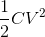5.Now let us increase the voltage by a small amount let it be dv due to this there will be displacement of plate let the displacement be dx. So work done against the spring force due to displacement of  plate B be F.dx.  Relation between current and applied voltage is given by,6. Now the input energy from this value of electric current is given by,

7. Now the change in this stored energy is given by,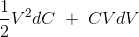8. Now apply principle of energy conservation by neglecting the higher order terms in the expression.

Input energy to the system = increase in the stored energy of the system + mechanical work done by the system.

By substituting all the values we get,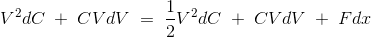Now the equation of force from the above equation is given by,### Torque equation of rotatory type electrostatic instruments:

Let us see in detail about torque equation of rotatory type electrostatic instruments.

Observe the following diagram.

1. By replacing F, dx in equation (1)  by Td , dA respectively we get deflecting torque of rotary type electrostatic instruments.

2. So the deflecting torque is given by,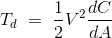3.At steady state we have controlling torque is given by, Tc = K × A. Where A is the deflection and it is given by,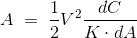As the deflection is directly proportional to square of voltage we have non- uniform scale.

Hence we have derived  torque equation of  electrostatic type instruments i.e for liner type electrostatic instruments and  rotatory type electrostatic instruments.

Generally electrostatic type instruments are used for measuring high voltages.

The main advantage of using electrostatic type instruments as voltmeters is we can extend the range of voltage that is to be measured.

### Methods to extend the range of voltage to be measured for electrostatic instruments:

1. Resistance potential dividers.

2.Capacitor multiplier technique.

### Resistance potential dividers to extend the range of voltage to be measured for electrostatic instruments:

Now let us see how to extend the range of voltage to be measured by using resistance potential dividers.

To understand it see the below circuit.

### Circuit diagram of resistance potential dividers to extend the range of voltage to be measured for electrostatic instruments:

The following diagram shows the circuit to extend the range of voltage to be measured by
electrostatic instruments using resistance potential dividers.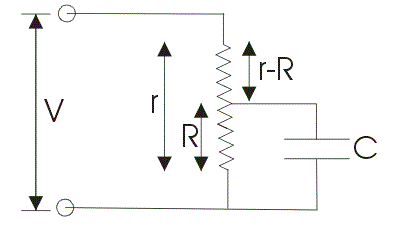### Procedure to extend the range of voltage to be measured by electrostatic instruments using resistance potential dividers:

1. Across r which is total resistance apply the voltage which is to be measured.

2. Across R which is a part of total resistance r connect an electrostatic capacitor.

3.Make one assumption that the capacitor which is connected is having infinite leakage resistance in case if we apply dc voltage. Here the multiplying factor is ratio of resistances i.e, r/R. Multiplying factor in ac case is same as dc case.

### Capacitor multiplier technique to extend the range of voltage to be measured for electrostatic instruments:

Now let us see how to extend the range of voltage to be measured by electrostatic instruments
using capacitor multiplier technique.

To understand it see the below circuit.

### Circuit diagram of capacitor multiplier technique to extend the range of voltage to be measured for electrostatic instruments:

The following diagram shows the circuit to extend the range of voltage to be measured by electrostatic instruments using capacitor multiplier technique.

### Procedure to extend the range of voltage to be measured by electrostatic instruments  using capacitor multiplier technique:

Let us calculate the multiplying factor.

1. From diagram we have series combination of capacitors. The equivalent capacitance is given by

2. Voltmeter impedance is given by Z1 = 1/jωC1 . Now total impedance is given by,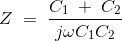3.Multiplying factor is given by,

Z/Z1 = 1 + C2 / C1.

In this way we can extend the range of voltage to be measured by electrostatic instruments with the help of  resistance potential dividers and capacitor multiplier technique.

### Advantages of electrostatic type instruments:

1. As the deflection torque is directly proportional to square of voltage we can measure both a.c and d.c voltages by using electrostatic type instruments.

2.High values of voltage can be measured  using electrostatic type instruments.

3. Current drawn by electrostatic type instruments  is low so power consumption of electrostatic type instruments is low.

### Disadvantages of electrostatic type instruments:

1.Electrostatic type instruments have non uniform scale.

2.Electrostatic type instruments are larger in size.

3.Electrostatic type instruments are costlier compared to other type of instruments.

4.Various operating forces present in electrostatic type instruments are small in magnitude.

Today we have learnt working principle, construction , torque equation and extending range of electrostatic type instruments.

You can download this article about working principle, construction , torque equation and extending range of electrostatic type instruments as PDF here.

## Differences Between Instrument and Power Transformers

In this article differences between power transformer and instrument transformer are discussed.Current transformer and Potential transformers come under instrument transformers.

### Differences Between Instrument and Power Transformers

Power Transformers Instrument Transformers
1. Mainly used to change voltage levels in
a power system.
1. Mainly used to extend the ranges of the
instruments while measuring parameters like
voltage,current,power etc.
2. They are required to transform huge amount
of power to the load.
2. They are required to transform very small power
as their loads are generally delicate moving elements
of the instruments.
3. They can be used to step up or step down the
voltage.
3. They are basically step down transformers and
used along with devices such as protective
relays,indicators etc.
4. The exciting current is a small fraction of the
secondary winding of load current.
4. As the load it self is small, the exciting current is
of the order of the secondary winding.
5. The cost is main consideration in the design
while efficiency and regulations are secondary
considerations.
5. Accuracy is the main consideration while designing
to keep ratio and phase angle errors minimum.
Cost is the second consideration.
6. As they handle large power, the heat
dissipation is the major consideration and
cooling method is necessary.
6. The power output is very small as loads are light.
Hence heating is not severe.
7. The limitation on the load is due to temperature
issue.
7. Accuracy is the main load limitation factor and not
the temperature rise.
8. Example : Distribution transformer used in
transmission.
8. Example : Current transformer and Potential
transformer

Tags:
difference between power transformer and current transformer
difference between power and potential transformer
instrument transformer pdf
how current transformers work
difference between current transformer and potential transformer
difference between power transformer and distribution transformer
difference between current and voltage transformer pdf
what is power transformer